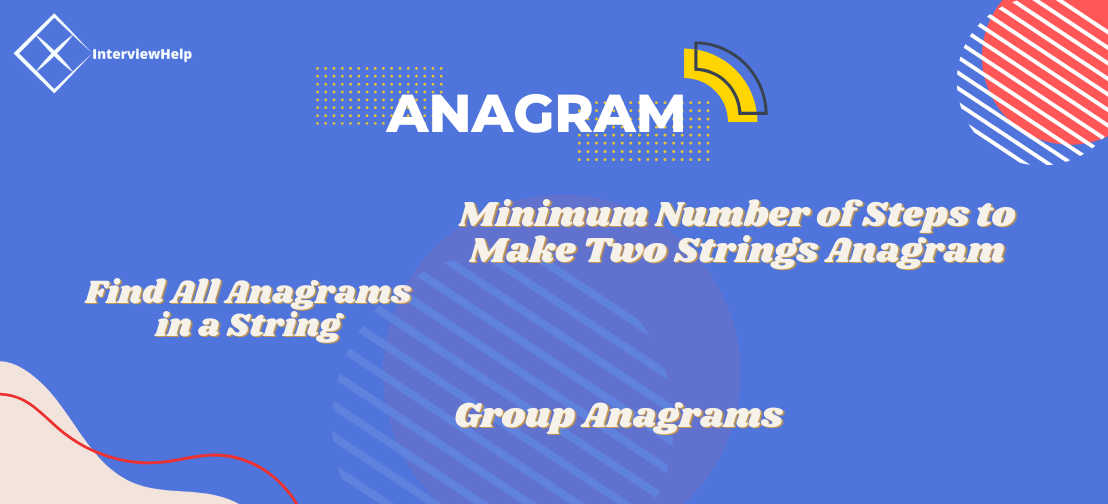# Anagram### Anagram

An anagram is a word formed by rearranging the letters of another word, using all the original letters exactly once. For example, the word anagram itself can be rearranged into nagaram, also the word binary into brainy and the word adobe into abode.

#### Valid Anagram

Given two strings `s` and `t`, how do we check whether they are anagrams of each other?

One way is to sort both strings lexicographically and compare the sorted strings:

``````def is_anagram(s, t):
return sorted(s) == sorted(t)
``````

This runs in `O(nlogn)` time and `O(n) space`.

Can we do better? If the letters in `s` and `t` are lowercase English letters, then we can simply store the frequency of each letter.

``````from collections import Counter

def is_anagram(s, t):
return Counter(s) == Counter(t)
``````

Or

``````def is_anagram(s, t):
frequency =  * 26
for s_char in s:
frequency[ord(s_char)-ord('a')] += 1
for t_char in t:
frequency[ord(t_char)-ord('a')] -= 1
return all(c == 0 for c in frequency)
``````

The last two frequency-based implementations have linear time complexity and constant space complexity.

### Minimum Number of Steps to Make Two Strings Anagram

Given two equal-size strings `s` and `t`. In one step you can choose any character of `t` and replace it with another character.

Return the minimum number of steps to make `t` an anagram of `s`.

### Solution:

``````def min_steps(s, t):
frequency =  * 26
for s_char in s:
frequency[ord(s_char) - ord('a')] += 1
for t_char in t:
frequency[ord(t_char) - ord('a')] -= 1
return sum(max(0, c) for c in frequency)
``````

### Find All Anagrams in a String

Given two strings `s` and `p`, return an array of all the start indices of `p`'s anagrams in `s`.

### Solution:

``````def find_anagrams(s, p):
p_counts =  * 26
for char in p:
p_counts[ord(char) - ord('a')] += 1

current_counts =  * 26
for i in range(-len(p)+1, len(s)-len(p)+1):
if i > 0:
current_counts[ord(s[i-1]) - ord('a')] -= 1
current_counts[ord(s[i+len(p)-1]) - ord('a')] += 1
if current_counts == p_counts:
yield i
``````

### Group Anagrams

Given an array of strings `strs`, group the anagrams together.

### Solution:

``````from collections import defaultdict

def group_anagrams(strs):
anagrams = defaultdict(list)
for string in strs:
anagrams[tuple(sorted(string))].append(string)
return list(anagrams.values())
``````